# A Novel Unsteady Model of Predicting the Productivity of Multi-fractured Horizontal Wells

A Novel Unsteady Model of Predicting the Productivity of Multi-fractured Horizontal Wells

Fanhui ZengChuan Long Jianchun Guo

State Key Laboratory of Oil and Gas Reservoir Geology and Exploitation, Southwest Petroleum University

Corresponding Author Email:
zengfanhui023024@126.com
Page:
117-124
|
DOI:
http://dx.doi.org/10.18280/ijht.330415
|
Accepted:
|
Published:
31 December 2015
| Citation

OPEN ACCESS

Abstract:

Forecasting the productivity of hydraulic fractured horizontal wells precisely is vital to develop low permeability gas and oil fields. The presented paper is based on the instantaneous point source function and potential superposition principle, considering the non-uniform flow distribution and the variable mass linear flow in the fractures of a fractured horizontal well. In the study a dynamic model of couple reservoir seepage and the fluid flow in fractures was built using a spatial-time discretization technique, and a solution method was also introduced. The results revealed that when regarding the inflow to the fracture as uniform and neglecting the pressure drop in the fractures, there may be huge deviation in the productivity calculation of a fractured horizontal well. Neglecting the pressure drop in the fractures may lead to a relatively high calculated value as compared to the actual production. The flow exhibited as an unsteady flow in the early stage, with the fracture segments which were closer to the horizontal wellbore showing a higher flow flux, while others had a lower flow rate. As producing time increase, the hydraulic fracturing horizontal well came to a pseudo-steady state phase, the flow distribution in the fractures tended to be uniform and steady. The daily output of hydraulic fractured horizontal well increases as fracture conductivity increases and there is optimized fracture conductivity consistent with the reservoir permeability.

Keywords:

Fractured horizontal well, Transient state, Point source function, Superposition, Flow distribution.

1. Introduction

In general, assuming different fracture geometries may lead to different fracture flow rates. If the fracture is a long rectangle instead of radial flow, it would result in linear flow being dominant in the fracture. Because the estimation of rate is strongly affected by specific characteristics of certain fracture conductivity and half length, the production model should take into account the correct fracture geometry. Larsen [16-17] established a model of fractured horizontal wells in an unsteady state based on numerical integration of Laplace transformed point-source solutions for unbounded reservoirs in three dimensions. Laplace transformed solutions for uniform-flux fractures in unbounded 3D reservoirs are first developed. Raghavan  used vertical well fracture models [19-20] with uniform-flux or infinite-conductivity rectangular fractures and the fracture communicates with the wellbore over its entire height to approximate the pressure-transient responses of fractured horizontal wells. Wang , according to the principles of potential superposition and continuity, developed a coupling model of variable mass flow in fractures and percolation in reservoir, which was established under steady state conditions.

So far, former scholars have investigated the theoretical models based on the reservoir seepage coupling with artificial fracture flow, but all the above approaches may be considered to be less rigorous because the description of the fluid flow inside the fracture is not comprehensive, but is deduced whilst assuming the flux to be uniform along the fracture. In fact, the interference among the fractures would result in non-uniform flux distribution along the fractures. In this study, a general solution for the rate calculation of a horizontal well intersected by finite conductivity vertical fractures was presented. Based on non-steady flow theory, potential principles, superposition principle and time superposition principle, a new method of predicting the productivity of fractured horizontal wells with the consideration of the non-uniform flux distribution along the fracture is presented, which produces more reasonable results. The factors influencing the productivity and field applications are presented in this article also.

2. Assumptions

In order to set up the mathematical model, some proper assumptions should be made for the mathematical model:

(1) The oil reservoir is closed at the top and bottom. The infinite anisotropic reservoir has a constant height h, porosity φ, and permeability K. At the initial time t+=0, the pressure is uniform throughout the oil reservoir and is equal to pi .

(2) The fractured horizontal well locates in the center of oil reservoir (Figure 1). The well is parallel to the  y-axis with length L, the number of fractures is N. The fractures are perpendicular to wellbore and equally or unequally spaced from each other, the fractures length xf are equal or unequal with each other, and fully penetrate the reservoir. The width is w, length is xf and height is h in y, x and z directions respectively.

(3) Single-phased and compressible fluid flows in the oil reservoir. The compressibility coefficient ct and viscosity μ are constant. The pressure drop of the fluid flow in the horizontal well bore is ignored.

## 1.png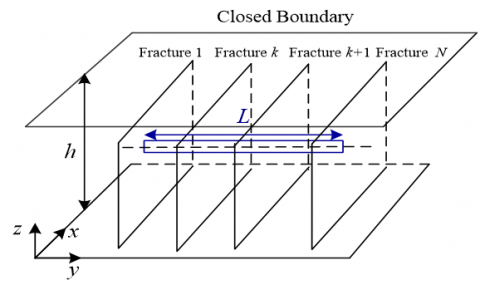Figure 1. The physical model of fractured horizontal well in an infinite tight oil reservoir

3. Mathematical Model

3.1 Reservoir seepage model

Since the fractures fully penetrate the reservoir, the hydraulic horizontal well fractures’ flow system can be simplified as a radial flow within the plane reservoir, and each fracture can be considered as being composed of microcline sinks points[14,22-23] .For convenience, the two monoplanes of the fracture are divided into ns equal segments and with a length of $\Delta x_{f k}=x_{f k} / n s$ , each portion is considered as one sink point as shown in Figure 2.

## 2.png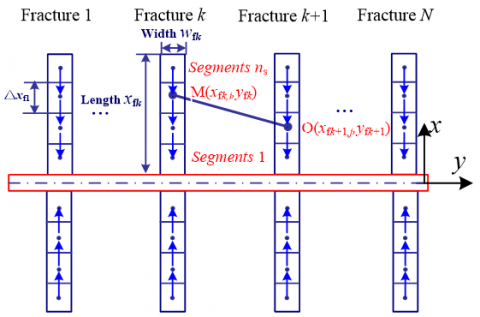Figure 2.  The sectional schematic of the fracture segments in the infinite conductivity model

The formation pressure rearranges because of the appearance of the artificial fractures. The production of the fractured horizontal wells changes and keeps declining over the production duration. However, if the time interval section is taken as small as possible, the flow can be treated as constant. Under the Cartesian coordinates plane, based on the pressure drawdown equation of the flow with constant rate of an infinite large sink point, the pressure drawdown of the point $O\left(x_{\mathrm{f} k+1, j}, y_{\mathrm{fk}+1}\right)$ caused by sink points $M\left(x_{f k, i}, y_{f k}\right)$ is as follows:

$p_{i}-p_{f k+1 j}=\frac{q_{f k, i} \mu B}{4 \pi K h}\left\{-E i\left(-\frac{\left(x_{f k, i}-x_{f k+1, j}\right)^{2}+\left(y_{f k}-y_{f k+1}\right)^{2}}{4 \eta \Delta t}\right)\right\}$     (1)

Where: p_{\mathrm{i}} is the initial pressure of the oil reservoir, MPa; $q_{\text {fir } i}$ is the pressure of jth fracture segment in the k+1th fracture, MPa; $q_{f k, i}$ is the oil flow rate of the ith fracture segment in the kth fracture at t=0+;  B is the fluid volume factor; $\left(x_{\mathrm{f} k, i}, y_{\mathrm{f} k}\right)$ are the center coordinates of ith fracture segment in the kth fracture; $\left(x_{f k+1, j}, y_{f k+1}\right)$ are the center coordinates of jth fracture segment in the k+1th fracture; K is the permeability, D; h is the reservoir thickness, m ; $\eta$ is transmissibility to diffusivity, m2/ks; $\eta=K / \mu c \phi$ ; $\mu$ is the viscosity, mPa·s; $c$ is the total compressibility, MPa-1; and $\phi$ is the porosity, decimal number ; $\Delta t$ is the production duration time, ks.

When there are ns equal segments on the ith fracture, the total pressure drops from the ith fracture at time t and location $\left(x_{\mathrm{f} k+1, j}, y_{f k+1}\right)$ is given as:

$p_{\mathrm{i}}-p_{\mathrm{f} k+1, j}=\sum_{i=1}^{2 n s} \frac{q_{\mathrm{f} k, i} \mu B}{4 \pi K h}\left\{-E i\left(-\frac{\left(x_{\mathrm{f} k, i}-x_{\mathrm{f} k+1, j}\right)^{2}+\left(y_{\mathrm{f} k}-y_{\mathrm{f} k+1}\right)^{2}}{4 \eta \Delta t}\right)\right\}$     (2)

Based on the superposition principle of the potential, the interaction between segments can be taken into account by superposition of pressures in space.

$p_{\mathrm{i}}-p_{\mathrm{f} k+1 j}=\sum_{k=1}^{N} \sum_{i=1}^{2 n s} \frac{q_{\mathrm{f} k, i} \mu B}{4 \pi K h}\left\{-E i\left(-\frac{\left(x_{\mathrm{f} k, i}-x_{\mathrm{f} k+1, j}\right)^{2}+\left(y_{\mathrm{f} k}-y_{\mathrm{f} k+1}\right)^{2}}{4 \eta \Delta t}\right)\right\}$       (3)

3.2 Fracture flow model

Assuming a one-dimensional linear inflow along the fracture into the wellbore, we can compute the friction caused by the fluid when flowing within the fracture based on the planar Darcy flow (Figure 3). For example, to compute the pressure drop caused by the fluid flow within the fracture from the jth segment (point $O_{f k+1，j}$ ) of k+1th fracture to the well bore (point O_{0} ) the calculation is as shown on the following page .

## 3.png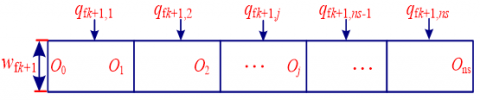Figure 3. Schematic representation of flow units within a fracture

$\Delta p_{\mathrm{f}, j-0}=\frac{\mu B}{2 k_{\mathrm{f}} w_{\mathrm{f} k+1} h} \Delta x_{\mathrm{f} k+1,1} q_{\mathrm{f} k+1,1}+\frac{\mu B}{2 k_{\mathrm{f}} w_{\mathrm{f} k+1} h}\left(\Delta x_{\mathrm{fr}+1,1}+\Delta x_{\mathrm{fr}+1,2}\right) q_{\mathrm{f} k+1,2}+\mathrm{L} +\frac{\mu B}{2 k_{\mathrm{f}} w_{\mathrm{f} k+1} h}\left(\Delta x_{\mathrm{f} k+1,1}+\Delta x_{\mathrm{f} k+1,2}+\mathrm{L}+\Delta x_{\mathrm{f} k+1, j}\right) q_{\mathrm{f} k+1 j}$

$+\frac{\mu B}{2 k_{\mathrm{f}} w_{\mathrm{f} k+1} h}\left(\Delta x_{\mathrm{f} k+1,1}+\Delta x_{\mathrm{f} k+1,2}+\mathrm{L}+\Delta x_{\mathrm{f} k+1, j}\right) q_{\mathrm{fk}+1, j+1}+\mathrm{L} +\frac{\mu B}{2 k_{\mathrm{f}} w_{\mathrm{f} k+1} h}\left(\Delta x_{\mathrm{f} k+1,1}+\Delta x_{\mathrm{f} k+1,2}+\mathrm{L}+\Delta x_{\mathrm{f} k+1, j}\right) q_{\mathrm{fk}+1, n s}$

$=\frac{\mu B}{2 k_{\mathrm{f}} w_{\mathrm{f} k+1} h}\left\{\sum_{i=1}^{j}\left(q_{\mathrm{f} k+1, i} \sum_{j=1}^{i} \Delta x_{\mathrm{f} k+1 j}\right)+\sum_{n=j+1}^{ns}\left[q_{\mathrm{f} k+1, n}\left(\sum_{i=1}^{j} \Delta x_{\mathrm{f} k+1, i}\right)\right]\right\}$      (4)

In the formula, $K_{{fk+1}}$ is the permeability of the k+1th fracture, D; w_{f k+1} is the width of the k+1th  fracture, m.

3.3 Transient reservoir-fracture coupled flow model

Due to the way in which the oil flow from the reservoir into the well bore can be separated into two different parts, Equation (3) and (4) have set up the formula correspondingly. The pressure node for each fracture segment is placed at the center of that segment, so the nodal pressure represents the reservoir pressure on the surface of the fracture that is in contact with the reservoir, the pressure continuity equation can then be established on the basis of Eq.(3) and (4). Since it has been assumed that the horizontal wellbore is infinitely conductive, the pressure is homogenous throughout the wellbore at any given time. In order to keep the pressure continuity in the oil reservoir and wellbore, Eq.(5) is established:

$p_{\mathrm{fk}+1, \mathrm{wf}}=p_{\mathrm{wf}}$    (5)

Where: p_{\mathrm{f} k+1, \mathrm{wf}} is the flow pressure of the well bottom, MPa;

Combining Eq.(3), (4) and (5) creates the reservoir-fracture coupled flow model of jth segment of the k+1th fracture as follows:

\begin{aligned} p_{\mathrm{i}}-p_{\text {wf }}=& \sum_{k=1}^{N} \sum_{i=1}^{2 \text { ns }} \frac{q_{\mathrm{f} k, i} \mu B}{4 \pi K h}\left\{-E i\left(-\frac{\left(x_{\mathrm{f} k, i}-x_{\mathrm{f} k+1, j}\right)^{2}+\left(y_{\mathrm{f} k}-y_{\mathrm{f} k+1}\right)^{2}}{4 \eta \Delta t}\right)\right\} +\frac{\mu B}{2 k_{\mathrm{f}} w_{\mathrm{f} k+1} h}\left\{\sum_{i=1}^{j}\left(q_{\mathrm{f} k+1, i} \sum_{j=1}^{i} \Delta x_{\mathrm{f} k+1, j}\right)+\sum_{n=j+1}^{n s}\left[q_{\mathrm{f} k+1, n}\left(\sum_{i=1}^{j} \Delta x_{\mathrm{f} k+1, i}\right)\right]\right\} \end{aligned}   (6)

There is no fluid flowing from other parts of the well bore except the fracture section, so the total production of the fractured horizontal well can be calculated as:

$Q=\sum_{k=1}^{N} \sum_{i=1}^{2 n s} q_{\mathrm{f} k, i}$    (7)

With a certain pwf, the model with Eq.(6) and (7) is able to calculate the flow rate of every fracture segment and fractured horizontal well.

At the time $\Delta t$ , we can write:

$\Delta p_{\mathrm{f}1,1}=q_{\mathrm{f}_{1,1}} F_{11,11}+q_{\mathrm{f1}, 2} F_{12,11}+q_{\mathrm{f1}, 3} F_{13,11}+\cdots+q_{\mathrm{fN}, 2 n s} F_{N 2 n s, 11}$     (8)

Where $\Delta p_{\mathrm{f1}, 1}=\left(p_{\mathrm{i}}-p_{\mathrm{f}{1}, 1}(x, y)\right)$ and gives the pressure drop at the pressure node of the 1st segment in the 1st fracture due to the flow from itself and all the other fracture segments. The location (x, y) is a point on the fracture circumference. The term $F_{N 2 n s, 11}$ in the above equation stands for the effect of 1st fracture segment on itself, where N, 2ns is the segment under consideration. Similar equations can be written for all other segments.

## 9.png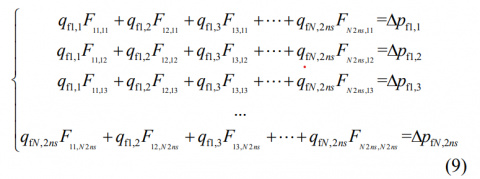Where:

$F_{k i, k+1 j}(\Delta t)=\frac{\mu B}{4 \pi K h}\left\{-E i\left(-\frac{\left(x_{\mathrm{f} k, i}-x_{\mathrm{f} k+1, j}\right)^{2}+\left(y_{\mathrm{f} k}-y_{\mathrm{f} k+1}\right)^{2}}{4 \eta \Delta t}\right)\right\}$

The unknowns in this problem are $N \times 2 n s$ number of q's at the nodes of the $N \times 2 n s$ segments. A total of $N \times 2 n s$ equations are needed to obtain a solution to the system of equations. By taking the pressure difference between wellbore and every other node, we can form $N \times 2 n s$ equations.  Because of the wellbore infinite conductivity assumption, pressure is constant in the well bore.

Solving the system of $N \times 2 n s$ equations given above, the q's from each node can be calculated for the first time step. By applying superposition in time, these solutions can be marched through time. It is assumed that all time steps are of equal length and are equal to $\Delta t$ . Therefore, for the jth segment of the k+1th fracture at $t=m \Delta t$ is:

\begin{aligned} p_{\mathrm{i}}-p_{\mathrm{f} k+1, j}(m \Delta t) &=\sum_{k=1}^{N} \sum_{i=1}^{2 n s}\left\{q_{\mathrm{f} k, i}(\Delta t) F_{k i, k+1 j}(m \Delta t)\right.+\left.\sum_{g=2}^{m}\left[q_{\mathrm{fk}, i}(g \Delta t)-q_{\mathrm{f} k, i}((g-1) \Delta t)\right] F_{k i, k+1 j}[(m-g+1) \Delta t]\right\} \end{aligned}     (10)

Solving the above set of equations, as was done for the first time step, can yield the flow rate distribution along all the fracture divisions at any time step. This procedure can be repeated for all the time steps.

4. Case Study and Sensitivity Analysis

A computer program was designed to test this model and compare with uniform flux and the infinite-conductivity fractured horizontal well mode. The basic parameter values of this case study are shown in Table 1.

Table 1. Basic parameters of a real example

 Parameter Data horizontal well length, Lf 400 m Reservoir Height, h 12m Permeability, K 0.0035 D Porosity, φ 10% Total Compressibility, ct 0.00035 MPa-1 Reservoir Initial pressure, pi 30 MPa Formation Volume Factor, B 1.084 Oil Viscosity, μ 8 mPa·s Oil Density at Reservoir Conditions, ρ 870 kg/m3 Bottom-hole pressure, pwf 25 MPa Well Diameter, rw 0.0889 m Fracture permeability, Kf 30 D Fracture width, w 5.0 mm Fracture length, xf 75 m Fracture numbers, N 4

The case study was run with the well bottom flow pressure constraint. Table 2 presents the fracture and daily production comparison with different models and the actual production. Model 1 is based on non-uniform flow distribution and infinite conductivity within the fracture, Model 2 is the proposed model, and Model 3 is based on uniform flow distribution and finite conductivity within the fracture[14,22].

Table 2. Comparison of Simulated solution and the actual productivity

 Parameters Productivity (m3/d) Model 1 Model 2 Model 3 Actual output 1 d 10 d 120 d 1 d 10 d 120 d 1 d 10 d 120 d 1 d 10 d 120 d Fracture 1 14.83 5.74 2.63 6.15 3.35 1.88 5.65 3.24 1.87 / / / Fracture 2 14.39 3.85 1.35 6.05 2.67 1.32 5.60 2.64 1.36 / / / Fracture 3 14.39 3.85 1.35 6.05 2.67 1.32 5.60 2.64 1.36 / / / Fracture 4 14.83 5.74 2.63 6.15 3.35 1.88 5.65 3.24 1.87 / / / Total 58.44 19.19 7.97 24.41 12.04 6.41 22.49 11.75 6.45 24.22 11.85 6.32

This result shows that the computational results with this proposed model which with non-uniform flux and finite conductivity is closer to the actual productivity. While Model 1 calculates the 1st day production is 58.44 m3/d, which far exceeded the actual value, this indicated that the impact of pressure loss within the fracture cannot be omitted. The results also show that the uniform flux case predicted less productivity than the non-uniform flux case. The reason for this is that in order to obtain the same flux at the center of the fracture as at its ends, for the uniform flux case, the fracture needs extra pressure drop to force some of the fluid to come to the center which would otherwise go to its ends. The ends of the fracture, being exposed to a larger portion of the reservoir than the center, gain access to reservoir fluids more easily.

4.1 Daily output of fracture and well with time

## 4.png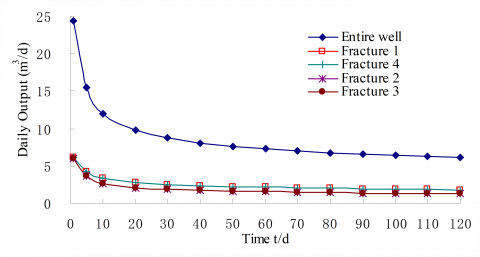Figure 4. Daily production over time

As shown as Figure 4, the daily output of fractured horizontal wells decreases rapidly from a high value at the beginning of production and then remained steady at a constraint of bottom hole pressure. This is because, in the early stage when a fractured horizontal well starts to produce, the pressure waves have not spread to most of the flow regions, and the fluid is merely flowing linearly from the bedrock around the fracture towards the fracture. When the pressure wave reaches the outer boundaries of the fractures, the flow enters the quasi-steady state with the production gradually tending toward steady output. Compared to the output of fractures in different positions, the flow rate is

approximately equal in each fracture at the beginning, and the gap between fractures is growing in the unsteady stage. The fracture output of the two ends (Fracture 1 and Fracture 4) is higher than the center (Fracture 2 and Fracture 3). That is, the outermost fractures have a larger drainage area and the inner fractures’ drainage areas exist in the interface between fractures. It also demonstrates that the fractures of the two ends have a protective screening effect on the middle fractures. So, an effective method of increasing production is to increase the fracture length of the two ends.

4.2 Flow rate distribution of different models

## 5.png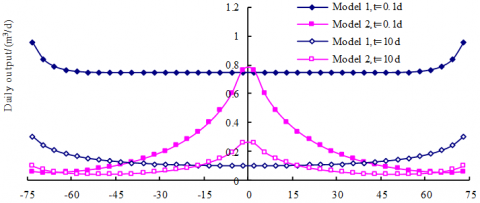Figure 5.  Flow rate distribution

The flux distribution along the discrete elements of Fracture 1 at different times (t = 0.1 d, t = 10 d) with Model 1 and Model 2 are shown in Figure 5. As Model 1 did not take into consideration the pressure drop within the fracture, the flux distribution at t = 0.1 d was a “U-shape”, with the flow at the two ends being relatively high. At t = 10 d, due to interference within the fractured segments there was an increase in strength, further strengthening the non-uniform flow distribution within the fracture. Model 2 considered the non-uniform flow rate distribution and finite conductivity along the fracture. The distribution of flow rate was wave-like in shape due to the influence of fractures. Therefore, the closer the fracture segment isto the wellbore, the lower the flow rate in the fracture segment. The flow rate of fractures is symmetrical around the wellbore.

In the early stage t=0.1 d, the pressure was uniform throughout the oil reservoir and was basically the original reservoir pressure. The pressure along the fracture dropped as the distance to the wellbore decreased, leading to a higher output of the fracture segment that was closer to the wellbore. As time increased (t = 10 d), the mutual interference within the fractured segments caused the flow distribution within the fracture to tend towards uniformity. However, the pressure was lowest at the junction of fracture with the wellbore, resulting in the flow distribution within the fracture displaying a “double U-shape”.

4.3 Flow rate distribution with time

## 6.png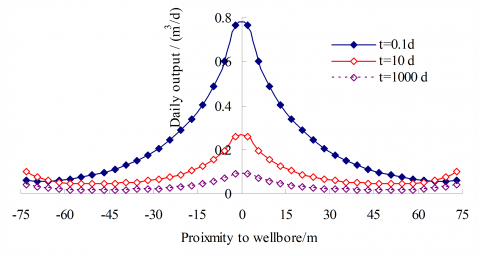Figure 6. Flow rate distribution with time

The flow distribution of Fracture 1 at different times is shown in Figure 6. Assuming the fracture has finite conductivity, there is pressure drop along the fracture. With the pressure dropping as the distance to the wellbore was reduced, the flow rate of these fracture segments increased. Over time (t = 10 d), the reservoir pressure wave gradually diffused outwards, which caused the flux of the intermediate

segments to gradually decrease, while that at the two ends of the fracture the flux gradually increased. During the stable production stage (t = 1000 d), the characteristics of uniform fluid production were exhibited along the entire length of the fracture.

4.4 Flow rate distribution with fracture conductivity

## 7.png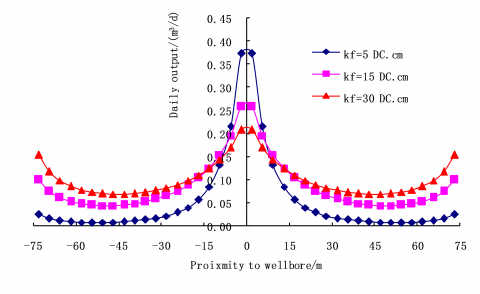Figure 7. Flow rate distribution with fracture conductivity

Figure 7 illustrates the relationship between the flux distribution with the conductivity of Fracture 1 at time t=10 d. It can be seen that the in-flow of fracture segments increased closer to the well bore. The lower the conductivity, the more non-uniform the flux distribution along the fracture. At t=10 d, the daily outputs of the 1st fracture were 2.15 m3/d, 3.44 m3/d, 4.09 m3/d with different fracture conductivity of 5 DC.cm, 15 DC.cm and 30 DC.cm respectively. This shows that the daily output increased rapidly at the beginning under lower fracture conductivity, and when the conductivity increased to a certain value, the change of fracture conductivity has little influence on the flow rate. So, it is necessary to optimize the fracture conductivity consistently with the reservoir supply capability.

5. Conclusions

(1)A reservoir/fractured horizontal well coupling model was established for finite conductivity considering the mutual interferences of fractures and segments under unsteady state conditions. The computational solution of the model was closer to the real output than previous models. Being a semi-analytical model, it does not suffer from numerical dispersion and grid sensitivities that result from numerical simulations.

(2)The analysis shows that the flow rate is approximately equal in each fracture at the beginning and the gap between fractures grew in the unsteady stage. The fracture output of the two ends was higher than that of the center because of interference. It is therefore an effective method of increasing production to increase the fracture length of the two ends.

(3)During the early non-steady state stage, the flux along the fracture exhibited a non-uniform characteristic: the flux increased as the fracture segment position moved closer to the well bore. As time increased, the mutual interference within the fractured segments caused the flow distribution within the fracture to tend towards uniformity.

(4)The daily output increased rapidly with the conductivity under lower fracture conductivity, and once the conductivity had increased to a certain value, the change of fracture conductivity had little influence on the flow rate, providing an optimal value for a certain formation.

Acknowledgement

This work was funded by the National Natural Science Foundation of China (project No.51525404,51504203), and by Open Fund (PLN1515) of State Key Laboratory of Oil and Gas Reservoir Geology and Exploitation (Southwest Petroleum University), a special fund from China’s central government for the development of local colleges and universities—the National First-level Discipline in the Oil and Gas Engineering Project (project No. 20150727), and Scientific Research Starting Project of Southwest Petroleum University (project No. 2014QHZ004).

References

1. Al-Kobaisi, M., Ozkan, E. and Kazemi, H., “A hybrid numerical/analytical model of a finite-conductivity vertical fracture intercepted by a horizontal well,” SPE Reservoir Evaluation & Engineering, 9(04): 345-355, 2006. DOI: 10.2118/92040-PA.

2. Medeiros, F., Ozkan, E. and Kazemi, H., “Productivity and drainage area of fractured horizontal wells in tight gas reservoirs,” SPE Reservoir Evaluation & Engineering, 11(05): 902-911, 2008. DOI: 10.2118/108110-MS.

3. Meyer, B.R., Bazan, L.W., Jacot, R.H. and Lattibeaudiere, M.G., “Optimization of Multiple Transverse Hydraulic Fractures in Horizontal Wellbores,” SPE Unconventional Gas Conference, Society of Petroleum Engineers, 2010. DOI: 10.2118/131732-MS.

4. Ozkan, E., Brown, M.L., Raghavan, R. and Kazemi, H., “Comparison of fractured-horizontal-well performance  in tight sand and shale reservoirs,”SPE Reservoir Evaluation & Engineering, 14(02): 248-259, 2011. DOI: 10.2118/121290-PA.

5. Giger, F., Reiss, L. and Jourdan, A., “The reservoir engineering aspects of horizontal drilling,” SPE Annual Technical Conference and Exhibition, Society of Petroleum Engineers, 1984. DOI: 10.2118/13024-MS.

6. Gringarten, A., Ramey Jr, H. and Raghavan, R., “Applied pressure analysis for fractured wells,” Journal of Petroleum Technology, 27(07): 887-892, 1975. DOI: 10.2118/5496-PA.

7. Joshi, S., 1987. “A review of horizontal well and drainhole technology,” SPE annual technical conference and exhibition, Society of Petroleum Engineers. DOI: 10.2118/16868-MS.

8. Mukherjee, H. and Economides, M.J., “A parametric comparison of horizontal and vertical well performance,” SPE Formation Evaluation, 6(02): 209-216, 1991. DOI: 10.2118/18303-PA.

9. Joshi, S., “Augmentation of well productivity with slant and horizontal wells” (includes associated papers 24547 and 25308), Journal of Petroleum Technology, 40(06): 729-739, 1988. DOI: 10.2118/15375-PA.

10. Prats, M., “Effect of vertical fractures on reservoir behavior-incompressible fluid case,” Society of Petroleum Engineers Journal, 1(02): 105-118, 1961. DOI: 10.2118/1575-G.

11. Raghavan, R. and Joshi, S.D., “Productivity of multiple drainholes or fractured horizontal wells,” SPE Formation Evaluation, 8(01): 11-16, 1993. DOI: 10.2118/21263-PA.

12. Wei, Y. and Economides, M.J., “Transverse hydraulic fractures from a horizontal well,” SPE Annual Technical Conference and Exhibition, Society of Petroleum Engineers, 2005. DOI: 10.2118/94671-MS.

13. Guo, B. and Schechter, D., “A simple and rigorous IPR equation for vertical and horizontal wells intersecting long fractures,” Journal of Canadian Petroleum Technology, 38(07), 1999. DOI: 10.2118/99-07-05.

14. Lei, Z., Cheng, S., Li, X. and Xiao, H., “A new method for prediction of productivity of fractured horizontal wells based on non-steady flow,” Journal of Hydrodynamics, Ser. B, 19(4): 494-500, 2007. DOI: 10.1016/S1001-6058(07)60145-0.

15. Lian, P., Cheng, L. and Cui, J., “A new computation model of fractured horizontal well coupling with reservoir,” International Journal for Numerical Methods in Fluids, 67(8): 1047-1056, 2011. DOI: 10.1002/fld.2407.

16. Larsen, L. and Hegre, T., “Pressure-transient behavior of horizontal wells with finite-conductivity vertical fractures,” International Arctic Technology Conference. Society of Petroleum Engineers, 1991. DOI: 10.2118/22076-MS.

17. Larsen, L. and Hegre, T., “Pressure transient analysis of multifractured horizontal wells,” SPE Annual Technical Conference and Exhibition, Society of Petroleum Engineers, 1994. DOI: 10.2118/28389-MS.

18. Raghavan, R., Chen, C.C. and Agarwal, B., “An analysis of horizontal wells intercepted by multiple fractures,” SPE/CIM/CANMET International Conference on Recent Advances in Horizontal Well Applications, Petroleum Society of Canada, 1994. DOI: 10.2118/HWC-94-39.

19. Cinco, L., Samaniego, V. and Dominguez, A., “Transient pressure behavior for a well with a finite-conductivity vertical fracture,” Society of Petroleum Engineers Journal, 18(04): 253-264, 1978. DOI: 10.2118/6014-PA.

20. Cinco-Ley, H. and Samaniego, F.v., “Transient pressure analysis for fractured wells,” Journal of petroleum technology, 33(9): 1749-1766, 1981. DOI: 10.2118/7490-PA.

21. Wang, Z., Jin, H. and Wei, J., “Interpretation of the coupling model between fracture variable mass flow and reservoir flow for fractured horizontal wells,” Journal of Hydrodynamics (Ser. A), 2: 010, 2009.

22. Jianchun, G., Qianli, L. and Fanhui, Z., “A productivity prediction model for a fractured well with wedge-shaped fractures,” Acta Petrolei Sinica, 34(2): 346-352, 2013.

23. Zeng, F., Guo, J., Xu, Y. and Zhao, J., “Factors affecting production capacity of fractured horizontal wells,” Petroleum Exploration and Development, 34(4): 474, 2007.

24. Jiali, G., Modern reservoir flow mechanics, Petroleum Industry Press, 2003.

25. Xiaoping, L., Underground Oil and Gas Seepage Mechanics, Beijing: Petroleum Industry Press, 2007.